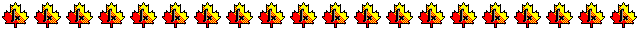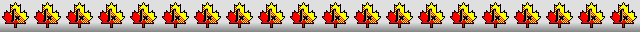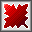Maple worksheets on the derivation of Runge-Kutta schemesNumerical methods topics:

They are all compatible with Classic Worksheet Maple 10.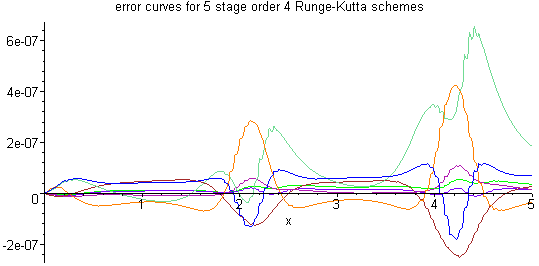Derivation of order 3 and 4 Runge-Kutta schemes using Taylor series - RKcoeff1.mws

• Derivation of a system of equations for calculating order 3 Runge-Kutta coefficients.
• The classical order 3 Runge-Kutta method.
• Derivation of a system of equations for calculating order 4 Runge-Kutta coefficients.
• The classical order 4 Runge-Kutta method.

Procedures related to the construction of Runge-Kutta schemes - RKcoeff_draw_tree.mws

• Procedures related to the construction and drawing of rooted trees along with their associated order conditions:
OrderConditionTrees, AllTrees, SimpleTrees, AllSimpleTrees, TreeOrderCondition,
TreeOrderCondition0
, TreeErrorTerm, TreeErrorCondition0, DrawTree.
• Procedures related to the construction of Runge-Kutta schemes via rooted trees:
OrderConditions, RowSumConditions, StageOrderConditions, PrincipalErrorConditions,
PrincipalErrorTerms
• These procedures are based on Maple code from "Runge-Kutta Methods, Trees, and Maple",
by Folkmar Bornemann, Selcuk Journal of Applied Mathematics, Vol. 2, No. 1, pp. 3-15, 2001.

Derivation of order 3 Runge-Kutta schemes - RKcoeff3.mws

• The classical order 3 scheme.
• Other examples.

Derivation of 4 stage, order 4 Runge-Kutta schemes - RKcoeff4a.mws

• The classical order 4 Runge-Kutta method.
• The 3/8 order 4 Runge-Kutta method.
• Gill's order 4 Runge-Kutta  method.
• Dormand's order 4 Runge-Kutta method.
• Other examples.

Derivation of 4 stage, order 4 Runge-Kutta schemes - RKcoeff4b.mws

• Ralston's method and two methods by King.
See: (1) "Runge-Kutta Methods with Minimum Error Bounds", by Anthony Ralston,
Mathematics of Computation, Vol. 16, 1962, pages 431 to 437.
(2) "Runge-Kutta Methods with Constrained Minimum Error Bounds", by Richard King,
Mathematics of Computation, Vol. 20, 1966, pages 386 to 391.
• Prince's 5 stage, order 4 Runge-Kutta method.
• Other examples.

Higher order Runge-Kutta schemes

The absolute stability region of an explicit Runge-Kutta method - RKcoeff_stability.mws

• Details concerning the stability region of an explicit Rune-Kutta method.
• The stability radius and the imaginary axis inclusion of a Runge-Kutta method.
• The procedure StabilityFunction can be used to construct the stability function of a an explicit Runge-Kutta method.

Evaluating order conditions of an explicit Runge-Kutta method using matrices - RKcoeff_matrix.mws

• The conversion procedure convert/Matrix_form enables an order condition to be evaluated by matrix multiplication.
This may be useful for some of the high order schemes.

Procedures related to the construction of Runge-Kutta schemes - butcher.zip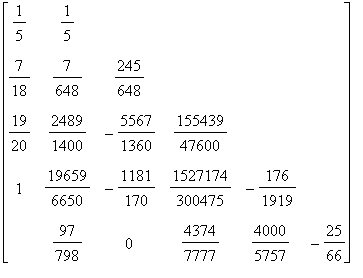Top of page

Main index